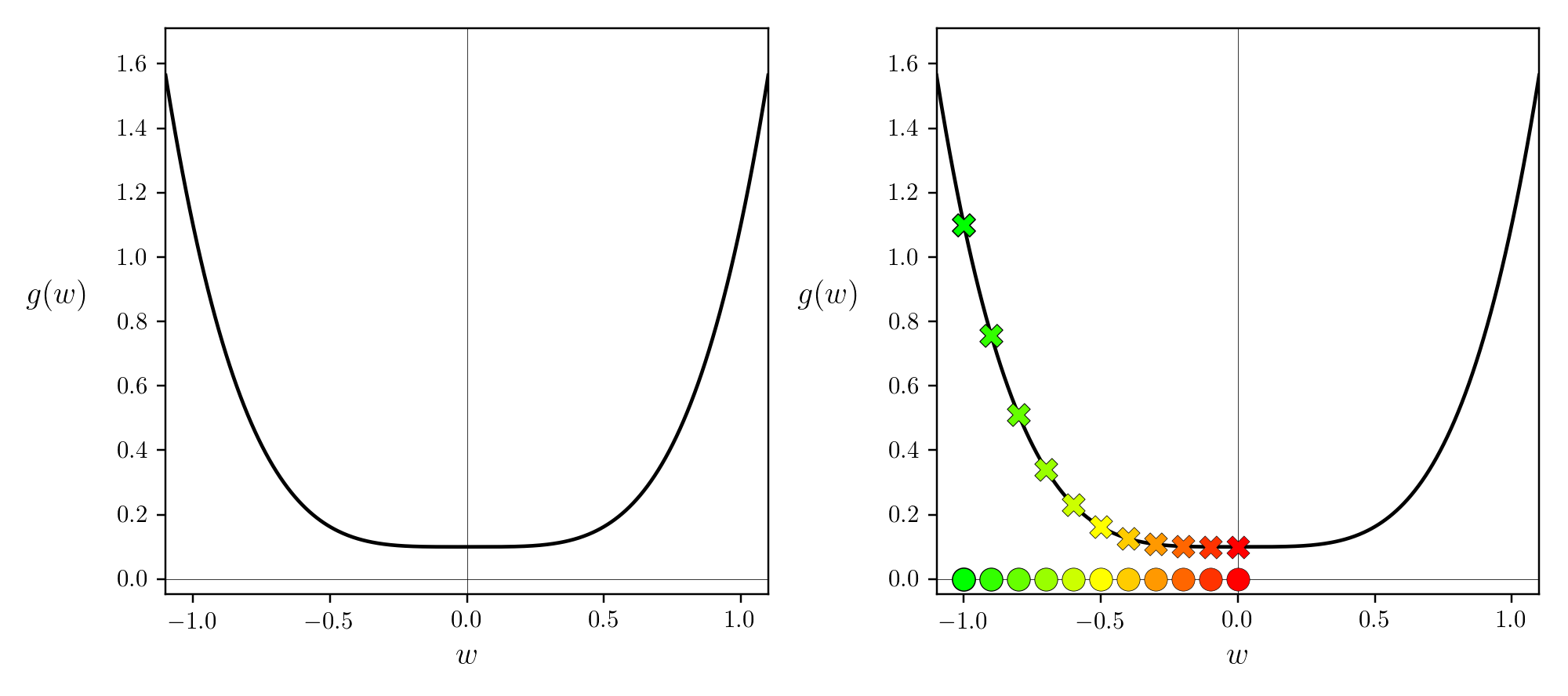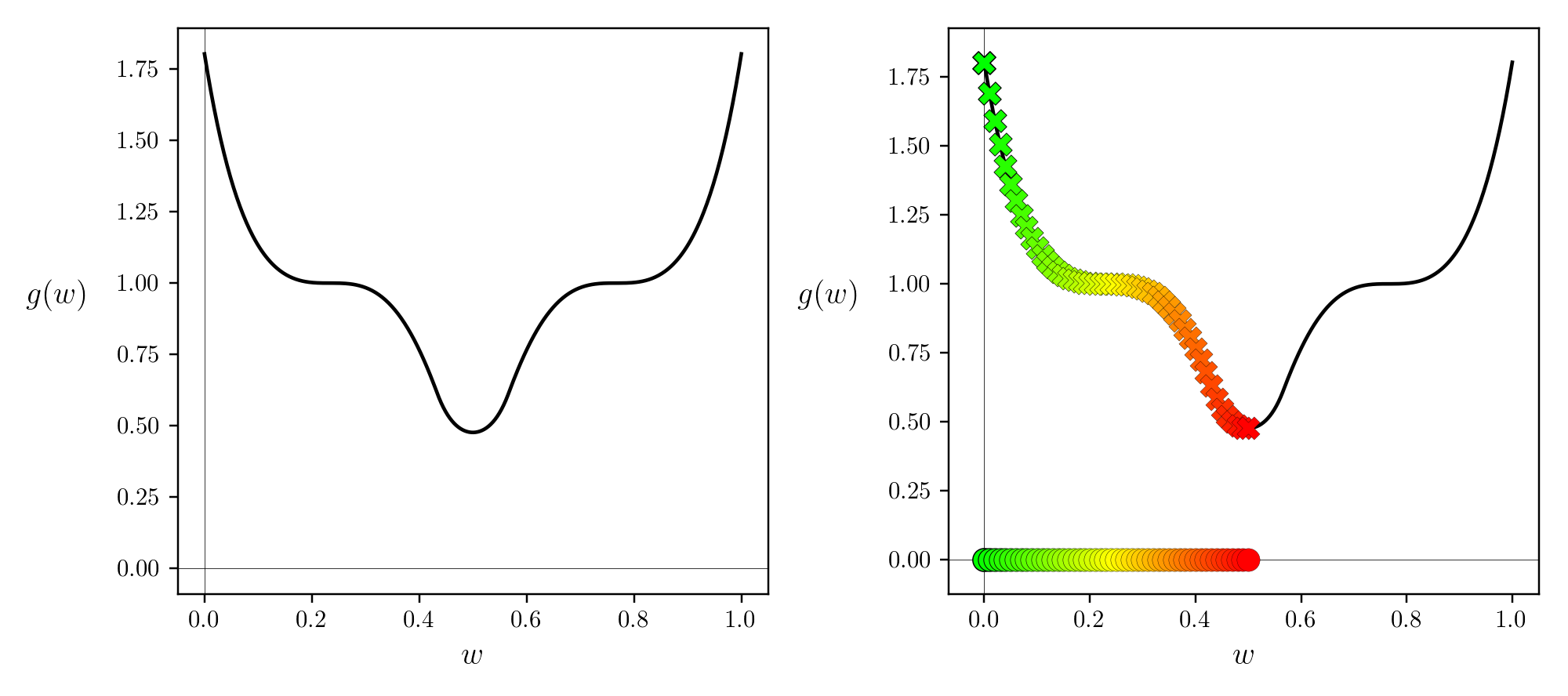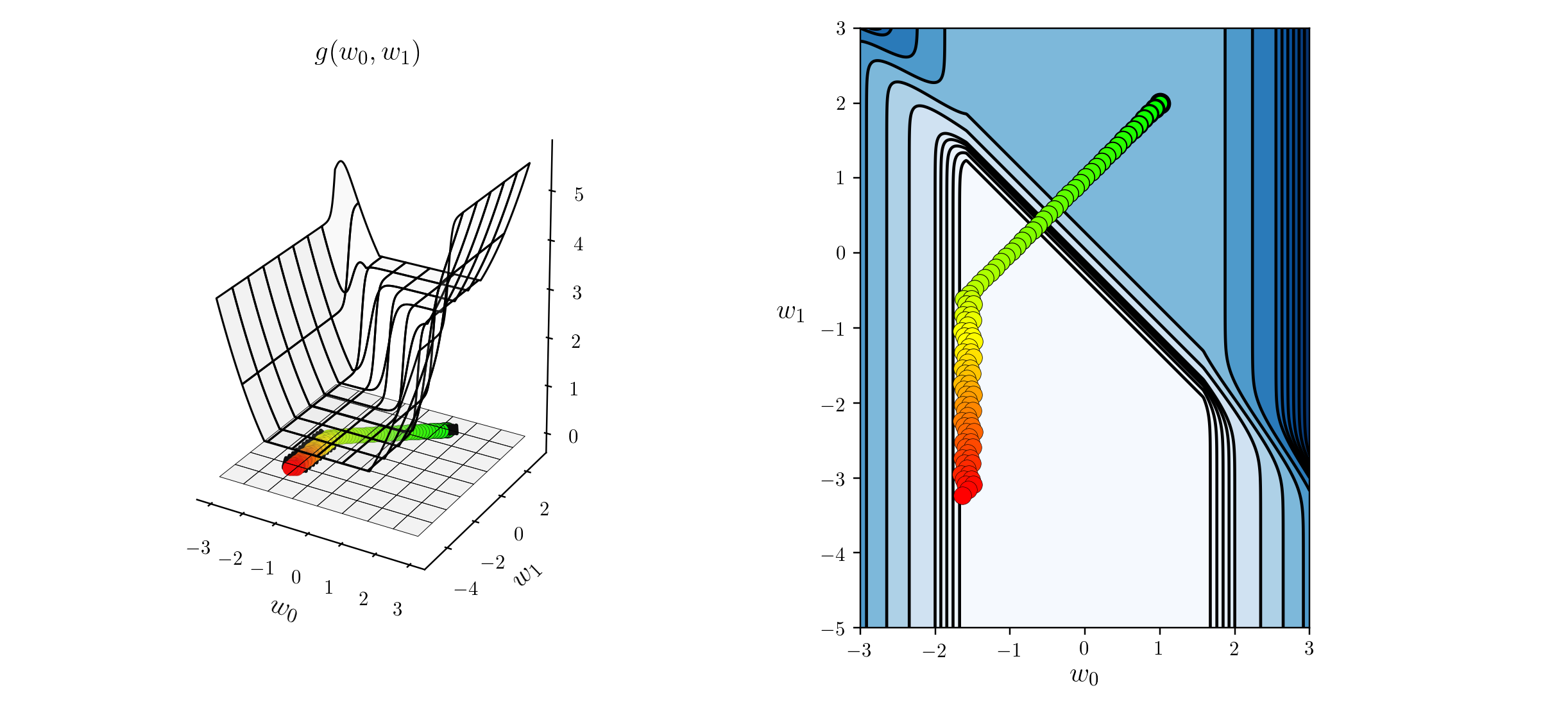* The following is part of an early draft of the second edition of Machine Learning Refined. The published text (with revised material) is now available on Amazon as well as other major book retailers. Instructors may request an examination copy from Cambridge University Press.

In the Section 3.7 we discussed a fundamental issue associated with the magnitude of the negative gradient and the fact that it vanishes near stationary points: gradient descent slowly crawls near stationary points which means - depending on the function being minimized - that it can halt near saddle points. In this Section we describe a popular enhancement to the standard gradient descent step, called normalized gradient descent, that is specifically designed to ameliorate this issue. The core of this idea lies in a simple inqiury: since the (vanishing) magnitude of the negative gradient is what causes gradient descent to slowly crawl near stationary points / halt at saddle points, what happens if we simply ignore the magnitude at each step by normalizing it out? In short by normalizing out the gradient magnitude we ameliorate some of the 'slow crawling' problem of standard gradient descent, empowering the method to push through flat regions of a function with much greater ease.

In :
In :

In Section 3.7 we saw that the length of a standard gradient descent step is proportional to the magnitude of the gradient or, in other words, the length of each step is not equal to the steplength / learning rate parameter $\alpha$ is given by

\begin{equation} \text{length of standard gradient descent step:} \,\,\,\, \alpha \, \Vert \nabla g(\mathbf{w}^{k-1}) \Vert_2. \end{equation}

As we saw it is precisely this fact that explains why gradient descent slowly crawls near stationary points, since near such points the gradient vanishes i.e, $\nabla g(\mathbf{w}^{k-1}) \approx \mathbf{0}$.

Since the magnitude of the gradient is to blame for slow crawling near stationary points, what happens if we simply ignore it by normalizing it out of the update step, and just travel in the direction of negative gradient itself? As we will see below there are several ways of going about doing this.

## Normalizing out the full gradient magnitude¶

One way to do this is by normalizing out the full magnitude of the gradient in our standard gradient descent step, and doing so gives a normalized gradient descent step of the form

\begin{equation} \mathbf{w}^{\,k} = \mathbf{w}^{\,k-1} - \alpha \frac{\nabla g(\mathbf{w}^{\,k-1})}{\left\Vert \nabla g(\mathbf{w}^{\,k-1}) \right\Vert_2 } \end{equation}

Doing this we do indeed ignore the magnitude of the gradient, since we can easily compute the length of such a step (provided the magnitude of the gradient is non-zero)

\begin{equation} \left\Vert \mathbf{w}^{\,k} - \mathbf{w}^{\,k-1} \right\Vert_2 = \left\Vert \left(\mathbf{w}^{\,k-1} - \alpha \frac{\nabla g(\mathbf{w}^{\,k-1})}{\left\Vert \nabla g(\mathbf{w}^{\,k-1}) \right\Vert_2} \right) - \mathbf{w}^{\,k-1} \right\Vert_2 = \left\Vert -\alpha \frac{\nabla g(\mathbf{w}^{\,k-1})}{\left\Vert \nabla g(\mathbf{w}^{\,k-1}) \right\Vert_2 }\right\Vert_2 = \alpha. \end{equation}

In other words, if we normalize out the magnitude of the gradient at each step of gradient descent then the length of each step is exactly equal to the value of our steplength / learning rate parameter $\alpha$

\begin{equation} \text{length of fully-normalized gradient descent step:} \,\,\,\, \alpha. \end{equation}

Notice then that if we slightly re-write the fully-normalized step above as

\begin{equation} \mathbf{w}^{\,k} = \mathbf{w}^{\,k-1} - \frac{\alpha}{{\left\Vert \nabla g(\mathbf{w}^{\,k-1}) \right\Vert_2 }} \nabla g(\mathbf{w}^{\,k-1}) \end{equation}

we can interpret our fully normalized step as a standard gradient descent step with a steplength / learning rate value $\frac{\alpha}{{\left\Vert \nabla g(\mathbf{w}^{\,k-1}) \right\Vert_2 }}$ that adjusts itself at each step based on the magnitude of the gradient to ensure that the length of each step is precisely $\alpha$.

Also notice that in practice it is often useful to add a small constant $\epsilon$ (e.g., $10^{-7}$ or smaller) to the gradient magnitude to avoid potential division by zero (where the magnitude completely vanishes)

\begin{equation} \mathbf{w}^{\,k} = \mathbf{w}^{\,k-1} - \frac{\alpha}{{\left\Vert \nabla g(\mathbf{w}^{\,k-1}) \right\Vert_2 } + \epsilon} \nabla g(\mathbf{w}^{\,k-1}) \end{equation}

Below we re-examine the examples of Section 3.7 where the slow-crawling problem of standard gradient descent was first diagnosed, only now we employ this fully-normalized gradient descent step.

#### Example 1. Ameliorating the slow-crawling behavior of gradient descent near the minimum of a function¶

Below we repeat the run of gradient descent first detailed in Example 5 of Section 3.7, only here we use a normalized gradient step (both the full and component-wise methods reduce to the same thing here since our function has just a single input). The function we minimize is

\begin{equation} g(w) = w^4 + 0.1 \end{equation}

whose minimum is at the origin $w = 0$. Here we use the same number of steps and steplength / learning rate value used previously (which led to slow-crawling with the standard scheme). Here however the normalized step - unaffected by the vanishing gradient magnitude - is able to pass easily through the flat region of this function and find a point very close to the minimum.

In :#### Example 2. Slow-crawling behavior of gradient descent near saddle points ameliorated via a normalized step¶

Here we illustrate how using a normalized descent step helps gradient descent pass easily by a saddle point of the function

\begin{equation} g(w) = \text{maximum}(0,(3w - 2.3)^3 + 1)^2 + \text{maximum}(0,(-3w + 0.7)^3 + 1)^2 \end{equation}

that would otherwise halt the standard gradient descent method. This function - which we saw in Example 6 of Section 3.7 - has a minimum at $w= \frac{1}{2}$ and saddle points at $w = \frac{7}{30}$ and $w = \frac{23}{30}$. Here we use the same number of steps and steplength / learning rate value used previously (which led to the standard scheme halting at the saddle point). Here however the normalized step - unaffected by the vanishing gradient magnitude - is able to pass easily through the flat region of the saddle point and reach a point of this function close to the mininum.

In :#### Example 3. Slow-crawling behavior of gradient descent in large flat regions of a function¶

Here we use the fully normalized step to minimize

\begin{equation} g(w_0,w_1) = \text{tanh}(4w_0 + 4w_1) + \text{max}(0.4w_0^2,1) + 1 \end{equation}

initializing our run on a large flat portion of this function. We saw this example previously in Example 7 of Section 3.7 where the standard method was unable to make virtually any progress at all due to the extreme flatness of the area in which we initialize. Here we use the and steplength / learning rate value used previously (which led to slow-crawling with the standard scheme), but only $100$ steps as opposed to the $1000$ used previously. Here however the normalized step - unaffected by the vanishing gradient magnitude - is able to pass easily through the flat region of this function and find a point very close to the minimum.

In :## Normalizing out the magnitude component-wise¶

Remember that the gradient is a vector of $N$ partial derivatives

\begin{equation} \nabla g(\mathbf{w}) = \begin{bmatrix} \frac{\partial}{\partial w_1}g\left(\mathbf{w}\right) \\ \frac{\partial}{\partial w_2}g\left(\mathbf{w}\right) \\ \vdots \\ \frac{\partial}{\partial w_N}g\left(\mathbf{w}\right) \\ \end{bmatrix} \end{equation}

with the $j^{th}$ partial derivative $\frac{\partial}{\partial w_j}g\left(\mathbf{w}\right)$ defining how the gradient behaves along the $j^{th}$ coordinate axis.

If we then look at what happens to the $j^{th}$ partial derivative of the gradient when we normalize off the full magnitude

\begin{equation} \frac{\frac{\partial}{\partial w_j}g\left(\mathbf{w}\right)}{\left\Vert \nabla g\left(\mathbf{w}\right) \right\Vert_2} = \frac{\frac{\partial}{\partial w_j}g\left(\mathbf{w}\right)}{\sqrt{\sum_{n=1}^N \left(\frac{\partial}{\partial w_n}g\left(\mathbf{w}\right)\right)^2}} \end{equation}

we can see that the $j^{th}$ partial derivative is normalized using a sum of the magnitudes of every partial derivative. If the $j^{th}$ partial derivative is already small in magnitude doing this will erase virtually all of its contribution to the final descent step. This can be problematic when dealing with functions containing regions that are flat with respect to only some of our weights / partial derivative directions, as it diminishes the contribution of the very partial derivatives we wish to enhance by ignoring magnitude.

Thus an alternative way to ignore the magnitude of the gradient is to normalize out the magnitude component-wise. So instead of normalizing each partial derivative by the magnitude of the entire gradient, we can normalize each partial derivative with respect to only itself. Doing this we divide off the magnitude of the $j^{th}$ partial derivative from itself as

\begin{equation} \frac{\frac{\partial}{\partial w_j}g\left(\mathbf{w}\right)}{\sqrt{\left(\frac{\partial}{\partial w_j}g\left(\mathbf{w}\right)\right)^2}} = \frac{\frac{\partial}{\partial w_j}g\left(\mathbf{w}\right)}{\left|\frac{\partial}{\partial w_j} g\left(\mathbf{w}\right)\right|} = \text{sign}\left(\frac{\partial}{\partial w_j}g\left(\mathbf{w}\right)\right) \end{equation}

So in the $j^{th}$ direction we can write this component-normalized gradient descent step as

\begin{equation} w_j^k = w_j^{k-1} - \alpha \, \frac{\frac{\partial}{\partial w_j}g\left(\mathbf{w}^{k-1}\right)}{{\sqrt{\left(\frac{\partial}{\partial w_j}g\left(\mathbf{w}^{k-1}\right)\right)^2}}}. \end{equation}

or equivalently as

\begin{equation} w_j^k = w_j^{k-1} - \alpha \, \text{sign}\left(\frac{\partial}{\partial w_j}g\left(\mathbf{w}^{k-1}\right)\right). \end{equation}

We can then write the entire component-wise normalized step can be similarly written as e.g.,

\begin{equation} \mathbf{w}^k = \mathbf{w}^{k-1} - \alpha \, \text{sign}\left(\nabla g\left(\mathbf{w}^{k-1}\right)\right) \end{equation}

where here the $\text{sign}\left( \cdot \right)$ acts component-wise on the gradient vector. We can then easily compute the length of a single step of this omponent-normalized gradient descent step (provided the partial derivatives of the gradient are all non-zero) as

\begin{equation} \left\Vert \mathbf{w}^{\,k} - \mathbf{w}^{\,k-1} \right\Vert_2 = \left\Vert \left(\mathbf{w}^{k-1} - \alpha \, \text{sign}\left(\nabla g\left(\mathbf{w}^{k-1}\right)\right)\right) - \mathbf{w}^{\,k-1} \right\Vert_2 = \left\Vert -\alpha \, \text{sign}\left(\nabla g\left(\mathbf{w}^{k-1}\right)\right) \right\Vert_2 = \sqrt{N} \, \alpha \end{equation}

In other words, if we normalize out the magnitude of gradient component-wise at each step of gradient descent then the length of each step is exactly equal to the value of our steplength / learning rate parameter $\alpha$

\begin{equation} \text{length of component-normalized gradient descent step:} \,\,\,\, \sqrt{N}\,\alpha. \end{equation}

Notice then that if we slightly re-write the $j^{th}$ component-normalized step $w_j^k = w_j^{k-1} - \alpha \, \frac{\frac{\partial}{\partial w_j}g\left(\mathbf{w}^{k-1}\right)}{{\sqrt{\left(\frac{\partial}{\partial w_j}g\left(\mathbf{w}^{k-1}\right)\right)^2} }}$ equivalently as

\begin{equation} w_j^k = w_j^{k-1} - \frac{\alpha}{\sqrt{\left(\frac{\partial}{\partial w_j} g\left(\mathbf{w}^{k-1}\right)\right)^2} } \, \frac{\partial}{\partial w_j}g\left(\mathbf{w}^{k-1}\right). \end{equation}

we can interpret our component normalized step as a standard gradient descent step with an individual steplength / learning rate value $\frac{\alpha}{\sqrt{\left(\frac{\partial}{\partial w_j} g\left(\mathbf{w}^{k-1}\right)\right)^2}}$ per component that all adjusts themselves individually at each step based on component-wise magnitude of the gradient to ensure that the length of each step is precisely $\sqrt{N} \, \alpha$. Indeed if we write

\begin{equation} \mathbf{a}^{k-1} = \begin{bmatrix} \frac{\alpha}{\sqrt{\left(\frac{\partial}{(\partial w_1} g\left(\mathbf{w}^{k-1}\right)\right)^2}} \\ \frac{\alpha}{\sqrt{\left(\frac{\partial}{\partial w_2} g\left(\mathbf{w}^{k-1}\right)\right)^2}} \\ \vdots \\ \frac{\alpha}{\sqrt{\left(\frac{\partial}{\partial w_N} g\left(\mathbf{w}^{k-1}\right)\right)^2}} \\ \end{bmatrix} \end{equation}

then the full component-normalized descent step can also be written as

\begin{equation} \mathbf{w}^{\,k} = \mathbf{w}^{\,k-1} - \mathbf{a}^{k-1} \odot \nabla g(\mathbf{w}^{\,k-1}) \end{equation}

where the $\odot$ symbol denotes component-wise multiplication. Again note that this is indeed equivalent to the previously written version of the full component-normalized gradient $\mathbf{w}^k = \mathbf{w}^{k-1} - \alpha \, \text{sign}\left(\nabla g\left(\mathbf{w}^{k-1}\right)\right)$. However note in practice if implemented as shown in equation (20) a small $\epsilon > 0$ is added to the denominator of each value of each entry of $\mathbf{a}^{k-1}$ to avoid division by zero.

#### Example 4. Minimizing a function with a flat region along only one dimension¶

In this example we use the function

\begin{equation} g(w_0,w_1) = \text{max}\left(0,\text{tanh}(4w_0 + 4w_1)\right) + \text{max}(0,\text{abs}\left(0.4w_0\right)) + 1 \end{equation}

to show the difference between fully and component normalizated steps on a function that has a very narrow flat region along only a single dimension of its input. Here this function - whose surface and contour plots can be seen in the left and right panels below respectively - is very flat along the $w_1$ direction for any fixed value of $w_0$, and has a very narrow valley leading towards its minima the $w_1$ dimension where $w_0 = 0$. If initialized at a point where $w_1 > 2$ this function cannot be minimized very easily using standard gradient descent or the fully-normalized version. In the latter case, the magnitude of the partial derivative in $w_1$ nearly zero everywhere, and so fully-normalizing makes this contribution smaller and halts progress. Below we show the result of $1000$ steps of fully-normalized gradient descent starting at the point $\mathbf{w}^0 = \begin{bmatrix} 2 \\ 2 \end{bmatrix}$, colored green (at the start of the run) to red (at its finale). As can be seen, little progress is made because the dircection provided by the partial derivative in $w_1$ could not be leveraged properly.

In :